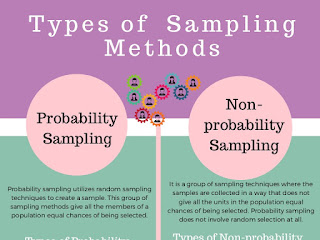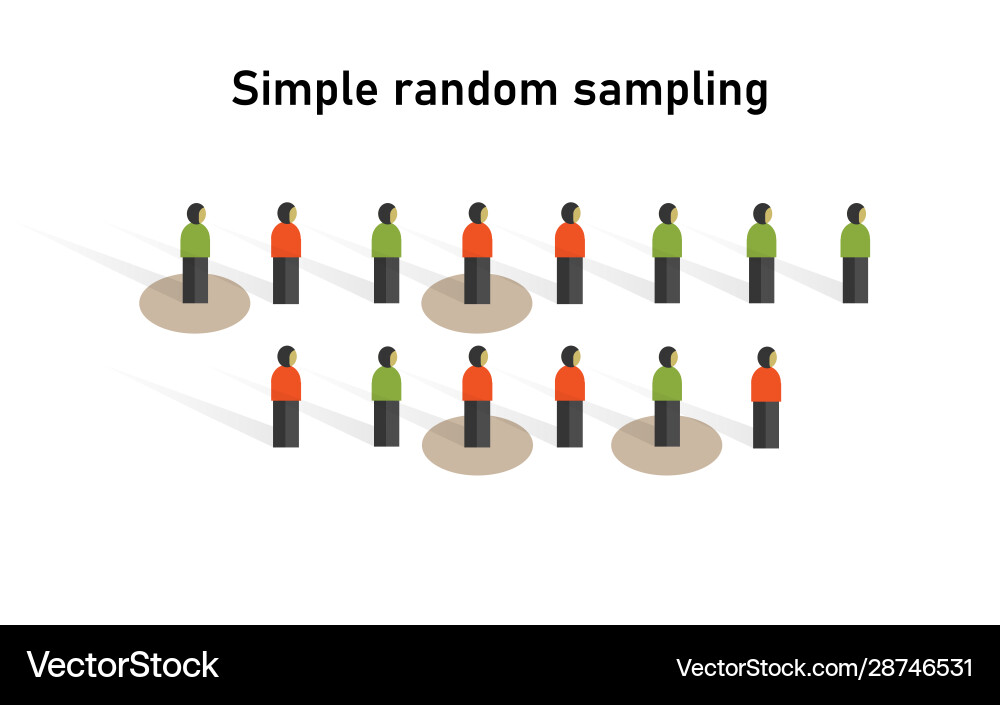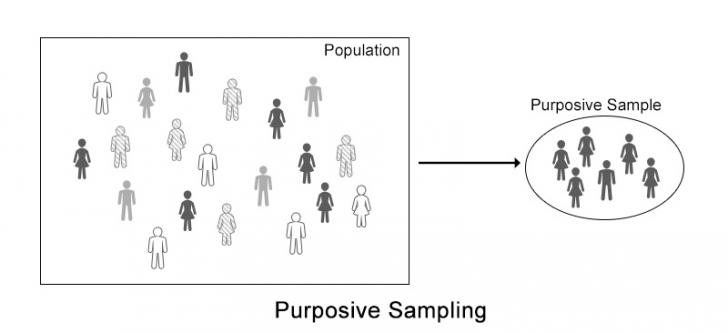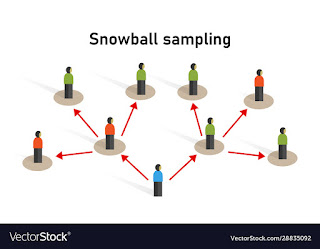# All You Need to Know About Sampling Distribution in Statistics

Sampling is a process of drawing a predetermined number of observations from a larger population. It is very difficult to make predictions on the population i.e. when our data is very huge so we must take samples and make a prediction on sample data which represents our population.
sample refers to a smaller, manageable version of a larger group. It is a subset containing the characteristics of a larger population. The good maximum sample size is usually around 10% of the population. eg) You want to know the literacy rate of India so it is very difficult to collect the data from each and every person from the country, so we will collect the samples randomly. It is one of the important tasks to determine a correct sample from the population.Entire Population

In this case, we must ensure that data is highly random and not taken on the basis of anyone ground like a particular state or gender-wise to avoid any bias towards one category.

## The sampling distributions are of two types:

### Probability Distribution:

In this distribution, with randomization, every element gets an equal chance to be picked up.

### Non-Probability Distribution:

In this distribution, every element does not get an equal chance to be selected.Type of Distributions

## Probability Distribution:

Probability sampling gives you the best chance to create a sample that is truly representative of the population. Using probability sampling for finding sample sizes means that you can employ statistical techniques like confidence intervals and margins of error to validate your results. There are various types of probability distribution sampling discussed below:

## a) Simple Random Sampling :

Simple Random Sampling is mainly used when we don’t have any prior knowledge about the target variable. In this type of sampling, all the elements have an equal chance of being selected.Simple Random Sampling
An example of a simple random sample would be the names of 50 employees being chosen out of a hat from a company of 500 employees. simple random sample is meant to be an unbiased representation of a group.

#### How you do simple random sampling?

1. Define the population.
3. List the population.
4. Assign numbers to the units.
5. Find random numbers.

## b) Systematic Sampling:

Here the elements for the sample are chosen at regular intervals of population. First, all the elements are put together in a sequence. Here the selection of elements is systematic and not random except the first element.

It is popular with researchers because of its simplicity. Researchers select items from an ordered population using a skip or sampling interval. For example, Saurabh can give a survey to every fourth customer that comes into the movie theatre.

#### How you do systematic sampling?

1. Calculate the sampling interval (the number of households in the population divided by the number of households needed for the sample)
2. Select a random start between 1 and sampling intervals.
3. Repeatedly add sampling interval to select subsequent households.

## c) Stratified Sampling:

In stratified sampling, we divide the elements of the population into strata (means small groups) based upon the similarity measure. All the elements are homogenous within one group and heterogenous from others.

### How you do stratified sampling?

1. Divide the population into smaller subgroups, or strata, based on the members’ shared attributes and characteristics.
2. Step 2: Take a random sample from each stratum in a number that is proportional to the size of the stratum.

• A stratified sample can provide greater precision than a simple random sample of the same size.
• Because it provides greater precision, a stratified sample often requires a smaller sample, which saves money.
For example, one might divide a sample of adults into subgroups by age, like 18–29, 30–39, 40–49, 50–59, and 60 and above.

#### The sample size for each strata (layer) is proportional to the size of the layer:

A sample size of the strata = size of the entire sample/population size * layer size.

## d) Cluster Sampling:

In one stage, the entire cluster is selected randomly for sampling. Here our entire population is divided into different clusters and then clusters are randomly selected.
In the second stage, here we first randomly select the clusters, combine those clusters and then randomly select samples from them.Cluster Sampling

How you do cluster sampling?
1. Estimate a population parameter.
2. Compute sample variance within each cluster (for two-stage cluster sampling).
3. Compute standard error.
4. Specify a confidence level.
5. Find the critical value (often z-score or a t-score).
6. Compute margin of error.
NOTE: Cluster sampling is less expensive and quicker.

## e) Multi-Stage Sampling:

Here, we can see the example where States are divided into districts further divided into villages and then households. In multi-stage sampling, the clusters are divided into groups and the groups are divided into subgroups until they cannot be further divided.Multi-Stage Sampling
How you do multi-stage sampling?
1. Choose a sampling frame, considering the population of interest.
2. Select a sampling frame of relevant separate sub-groups.
3. Repeat the second step if necessary.
4. Using some variation of probability sampling, choose the members of the sample group from the sub-groups.
Advantages: cost and speed. convenience (only need a list of clusters and individuals in selected clusters) usually more accurately than clusters for the same total size.

## 2) Non-Probability Distribution types:

Nonprobability sampling is a sampling technique where the odds of any member being selected for a sample cannot be calculated. Nonprobability sampling is defined as a sampling technique in which the researcher selects samples based on the subjective judgment of the researcher rather than random selection.

### Types of Non-Probability Sampling:Type of Non-Probability Sampling

## a) Convenience Sampling:

Convenience sampling which is also known as availability sampling is a specific type of non-probability sampling method. The sample is taken from a group of people easy to contact or to reach. For example, standing at a mall or a grocery store and asking people to answer questions would be an example of a convenience sample.Convenience Sampling
The relative cost and time required to carry out a convenience sample are small in comparison to probability sampling techniques. This enables you to achieve the sample size you want in a relatively fast and inexpensive way limitations include data bias and generating inaccurate parameters. Perhaps the biggest problem with convenience sampling is dependence. Dependent means that the sample items are all connected to each other in some way.

## b) judgment Sampling:

Judgment sampling is a common non-probability method. It is also called a purposive method. The researcher selects the sample based on the judgment. This is usually an extension of convenience sampling.Judgment Sampling

Judgment sampling may be used for a variety of reasons. In general, the goal of judgment sampling is to deliberately select units (e.g., individual people, events, objects) that are best suited to enable researchers to address their research questions. This is often done when the population of interest is very small, or desired characteristics of units are very rare, making probabilities sampling infeasible.

## c) Quota Sampling:

A sampling method of gathering representative data from a group. As opposed to random sampling, quota sampling requires that representative individuals are chosen out of a specific subgroup. For example, a researcher might ask for a sample of 50 females or 50 individuals between the ages of 32-43.

Quota sampling is used when the company is short of time or the budget of the person who is researching on the topic is limited. Quota sampling can also be used at times when detailed accuracy is not important. To create a quota sample, knowledge about the population and the objective should be well understood.

## d) Snowball Sampling:

As described in Leo Goodman’s (2011) comment, snowball sampling was developed by Coleman (1958-1959) and Goodman (1961) as a means for studying the structure of social networks.
Snowball sampling (or chain sampling, chain-referral, sampling referral sampling) is a non-probability sampling technique where existing study subjects recruited future subjects from among their acquaintances. Snowball sampling analysis is conducted once the respondents submit their feedback and opinions. Wsed where potential participants are hard to find.Snowball Sampling